# Comparison function

(diff) ← Older revision | Latest revision (diff) | Newer revision → (diff)

A function that is used in studying the character of growth of the modulus of an entire function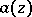when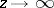; a comparison is normally made here between the behaviour of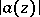and that of a certain "good" entire function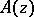. Here naturally arises the problem of describing a sufficiently broad set of entire functions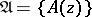the elements of which could successfully be used as "comparison standards" .

An entire function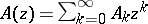is called a comparison function, or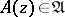, if: 1)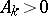(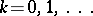); and 2)as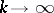. An entire functionis called-comparable if there exists a constant,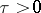, such that(1)

The lower boundof the numbersfor which the relation (1) is fulfilled is called the-type of the-comparable entire function. The following theorem on-types holds: If an entire functionis comparable with,, then its-typecan be calculated using the formula(2)

The given classof comparison functions is known to give a complete solution of this problem, since for any entire function, other than a polynomial, there exists a comparison function,, such thatis comparable withand such that its-type is equal to 1.

If an entire function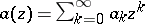is comparable with,, and its-type is equal to, then the function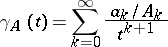is analytic, according to (2), for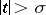; it is called-associated with. In this case, the generalized Borel representation holds for: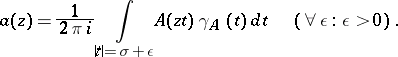(3)

If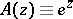is taken as a comparison function, then (3) is the classical Borel integral representation of entire functions of exponential type.

If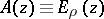holds in (3), where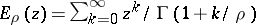() is a Mittag-Leffler function, then (3) is an integral representation for any entire functionof orderand of type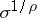(is the type ofin the classical sense).

For certain cases of, an inverse transformation to (3) has been constructed (see, for example , which has a bibliography relating to comparison functions). Comparison functions and the Borel representation (3) are used in various questions of analysis (see, for example, , ). If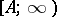is the class of entire functions that are comparable with a given comparison function, then, for any sequence of comparison functions, there always exists an entire functionsuch that.

How to Cite This Entry:
Comparison function. Encyclopedia of Mathematics. URL: http://encyclopediaofmath.org/index.php?title=Comparison_function&oldid=18614
This article was adapted from an original article by Yu.A. Kaz'min (originator), which appeared in Encyclopedia of Mathematics - ISBN 1402006098. See original article